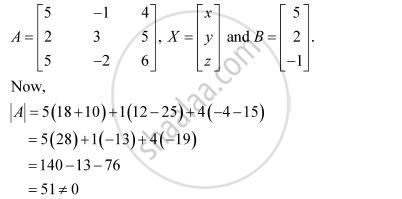Share

# Examine the Consistency of the System of Equations. 5x − Y + 4z = 5 2x + 3y + 5z = 2 5x − 2y + 6z = −1 - CBSE (Science) Class 12 - Mathematics

ConceptApplications of Determinants and Matrices

#### Question

Examine the consistency of the system of equations.

5x − y + 4z = 5

2x + 3y + 5z = 2

5x − 2y + 6z = −1

#### Solution

The given system of equations is:

5x − y + 4z = 5

2x + 3y + 5z = 2

5x − 2y + 6z = −1

This system of equations can be written in the form of AX = B, where∴ A is non-singular.

Therefore, A−1 exists.

Hence, the given system of equations is consistent.

Is there an error in this question or solution?

#### Video TutorialsVIEW ALL 

Solution Examine the Consistency of the System of Equations. 5x − Y + 4z = 5 2x + 3y + 5z = 2 5x − 2y + 6z = −1 Concept: Applications of Determinants and Matrices.
S